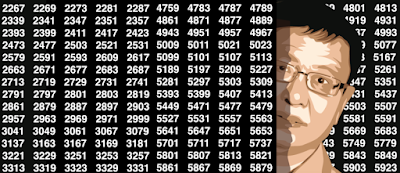## Sunday, August 30, 2020

### The Exponential Mean: Alternative to Classic Means

Given n observations x1, ..., xn, the generalized mean (also called power mean) is defined asThe case p = 1 corresponds to the traditional arithmetic mean, while p = 0 yields the geometric mean, and p = -1 yields the harmonic mean. See here for details. This metric is favored by statisticians. It is a particular case of the quasi-arithmetic mean

Here I introduce another kind of mean called exponential mean, also based on a parameter p, that may have an appeal to data scientists and machine learning professionals. It is also a special case of the quasi-arithmetic mean. Though the concept is basic, there is very little if any literature about it. It is related to the LogSumExp and the Log semiring. It is defined as follows:Here the logarithm is in base p, with p positive. When p tends to 0, mp is the minimum of the observations. When p tends to 1, it yields the classic arithmetic mean, and as p tends to infinity, it yields the maximum of the observations.• Advantages of the exponential mean
• Illustration on a test data set
• Illustration on a test data set
• Doubly exponential mean